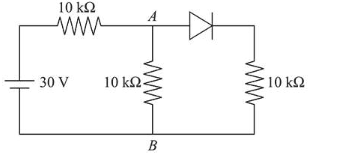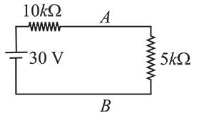# In the figure, potential difference between A and B is

Question:

In the figure, potential difference between $A$ and $B$ is:1. (1) $10 \mathrm{~V}$

2. (2) $5 \mathrm{~V}$

3. (3) $15 \mathrm{~V}$

4. (4) zero

Correct Option: 1

Solution:

(1) The given circuit has two $10 k \Omega$ resistances in parallel, so we can reduce this parallel combination to a single equivalent resistance of $5 \mathrm{k} \Omega$.Diode is in forward bias. So it will behave like a conducting wire.

$V_{A}-V_{B}=\frac{30}{5+10} \times 5=10 \mathrm{~V}$